﻿ Learn about Neural Network in Machine Learning: DZONE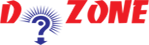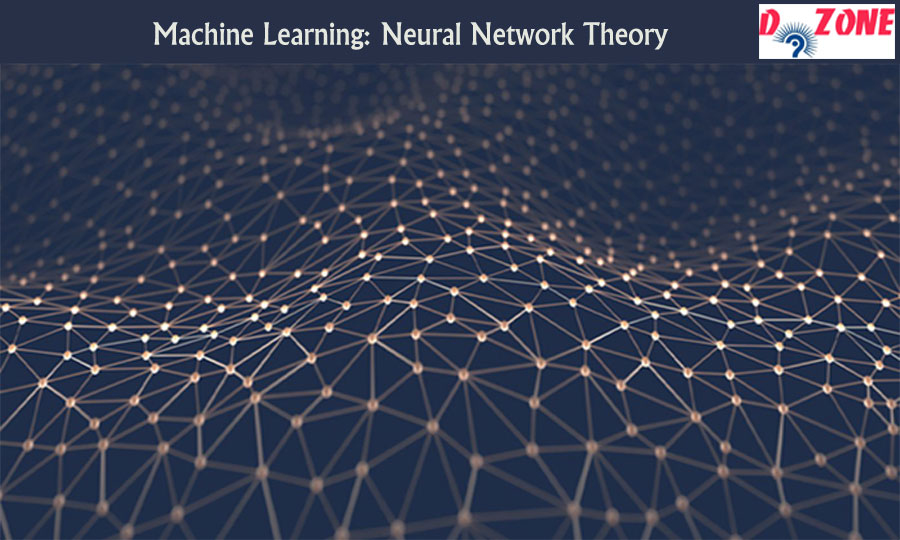# Learn Artificial Neural Network in Machine Learning

Cost-effective course for short period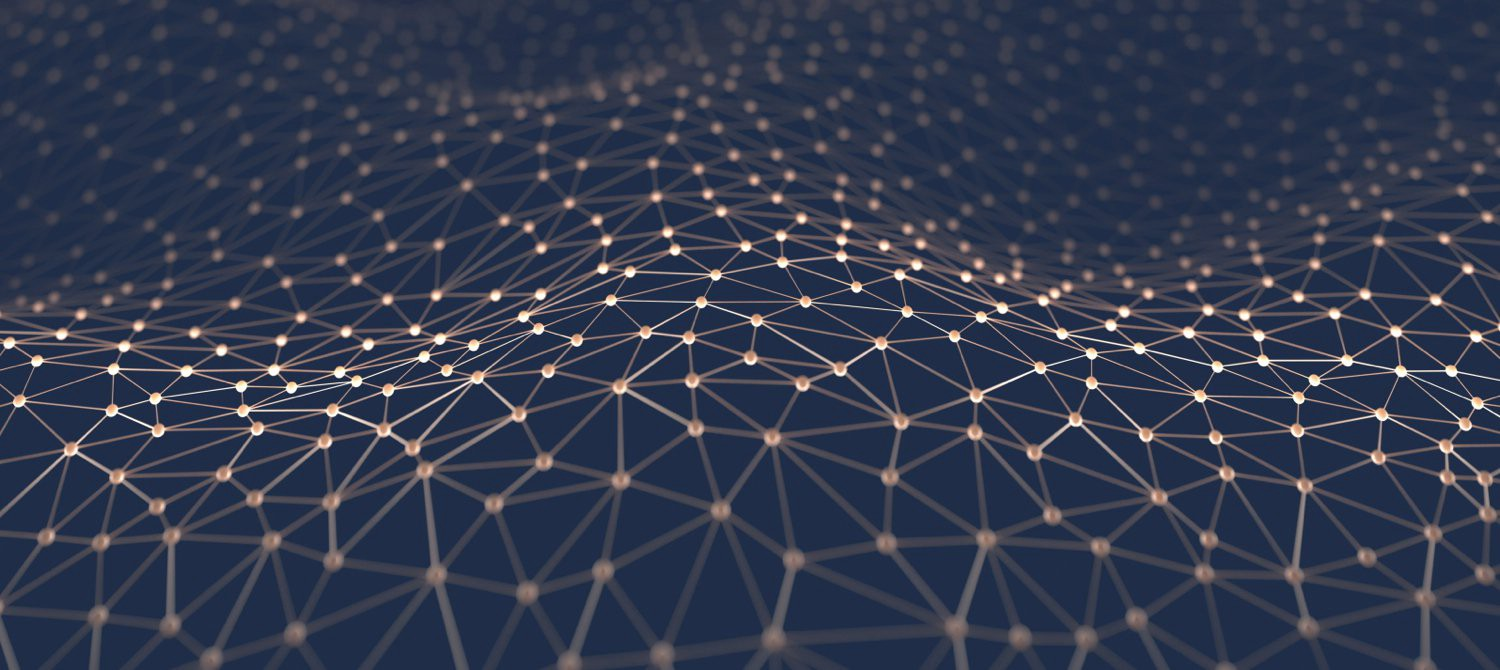Theory of Neural Network in Machine Learning

This article is for all the learners or enthusiasts who want to know about neural networks and are searching a perfect destination to start learning it in an easy way. So, without further due lets start the introduction about neural network theory.

What is a Neural Network?

Have you ever noticed your surroundings, there are numerous things that are working itself without any guidance or control by a human being. For instance, voice to text feature in smartphones, smart personal assistants like Siri, Google Now and Cortana, self-driven cars, security surveillance and so on and on. All these features has one thing in common and that is AI or Artificial Intelligence. In this blog, you will study about artificial neural network which is the foremost step for artificial intelligence.

Artificial Neural Network works as similar as the human brain works. Like in our brain, there are billions of neurons that can store all kind of information passes through it. These neurons, gives humans a power to think logically and also process huge amount of data daily without bothering us. All the neurons are connected to each other and pass the information from one to another. Likewise, artificial neural network works. the neural network in python is built by connecting so many artificial neurons or nodes. Each node in the network has particular amount of weight and bias value, the weight gets multiplied and bias gets added according to the input value. When the data passes through the network multiple times then it is called iteration or epoch. The input data gets updated with each epoch, weights and bias of neurons.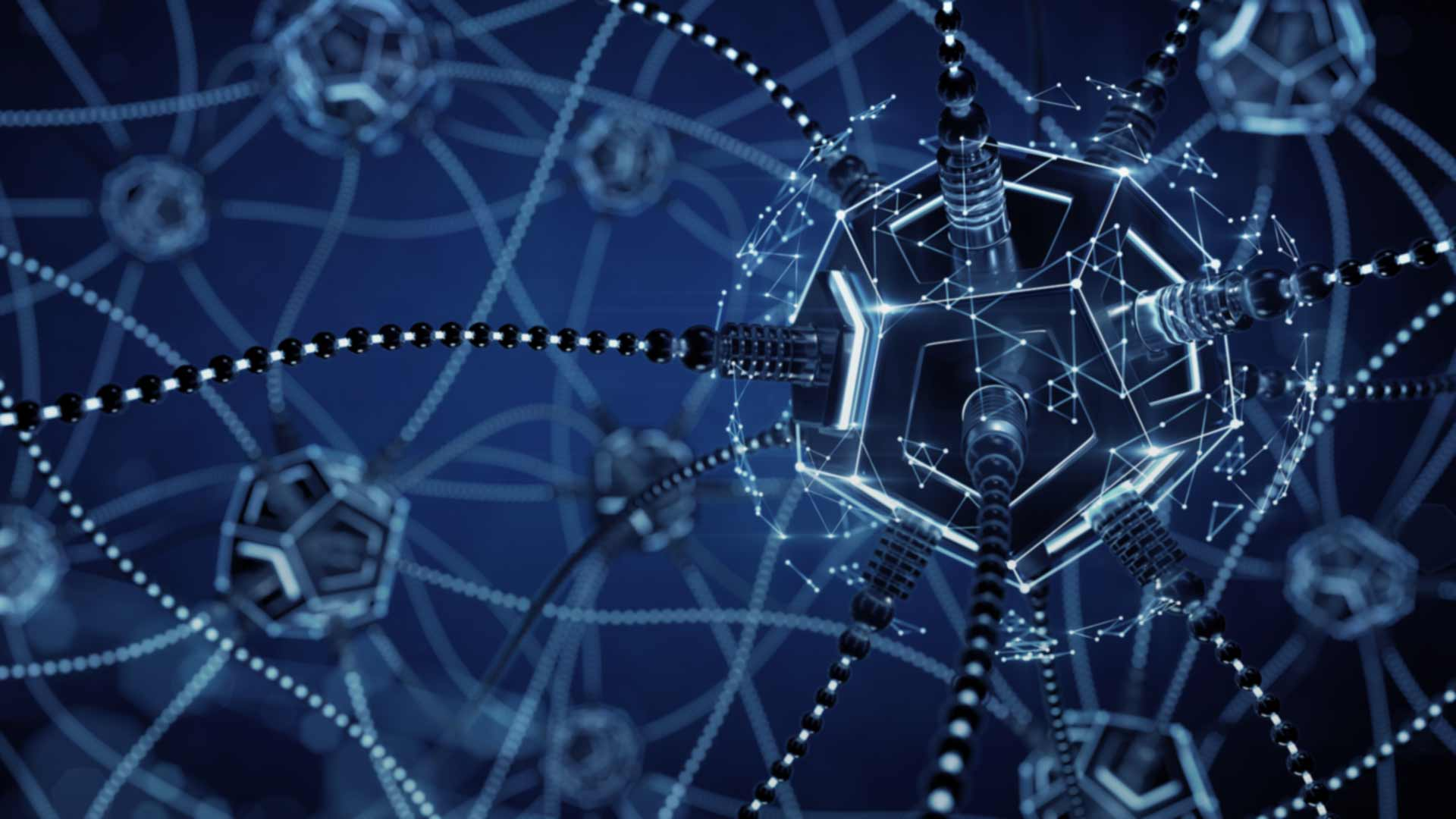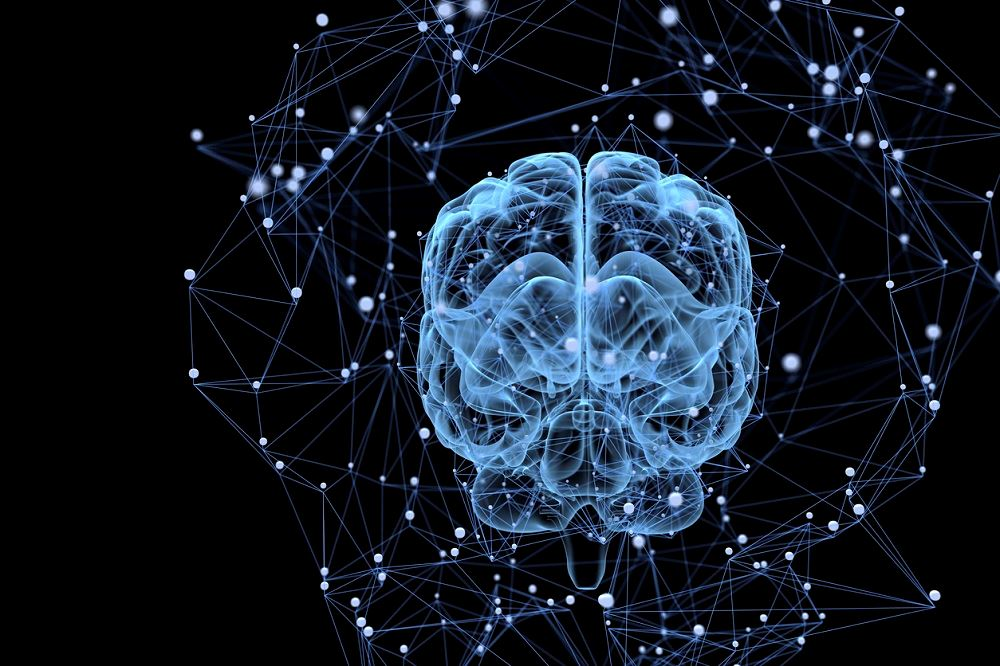Learning Process of Artificial Neural Network

The neural network is made up of so many neurons that are connected to each other. Each neuron has its particular weight which get multiplied by the input value and are inter-connected to each other. every node has an activation function that gives the output of the neuron. It is basically used to introduce non-linearity in the modeling capabilities of the network.

When the data reaches the output layer then it compares the expected output with the computer output. If their is any difference between the two then it is known as training loss of the network. If we find any training loss then the optimizer enters into it. The optimizer is a function in the neural network that tries to adjust the weights and bias of the neurons to reduce the loss of the network. Once the optimizer computes the loss, it try to change the weights of the hidden layer neurons in such a manner so that the loss can be minimized. After updation, it goes back to the previous layer and try to do the same. So, the optimized starts its work from the last layer and finish it at the first layer, this whole process is called as backpropogation.

To create a good network, we pass this data again and again through the network till the backpropogation finds the appropriate weights and bias for each and every neuron. So, this whole process is known as training the network. Once the network gets trained properly we can stop the training process and save the network. So, now you can use the saved network to predict the output of unseen data.

## Learn the difference between

### AI and Neural Network